# Science and Music

## Introduction

There are people who like pop music, those who like rock music, dance, classical or even a little of everything but, let's face it, most people love music in some form or another. How many, however, know what music actually is. This article will briefly try to address this by explaining the basic science behind music.

## Waves and Sound

If you are already know a little about physics then it may not be worth reading this section. However, if not, or you are just interested then please read on.

What is a wave? To answer this question imagine a horizontal row of particles. Now imagine that one of these particles is moving up and down about a central point i.e. it is oscillating. The particle moves from its central position up to a maximum position. It then starts to move down; it crosses its central position until a maximum and then moves back to the centre. This motion repeats. the maximum distance that it moves is known as its amplitude. This is shown in the figure below. The particle is shown at different times oscillating about a central point.A particle oscillating about a central point. Different points in the motion are shown with time. The amplitude is the maximum distance from the centre of its motion.

Now imagine that there is a particle to the left that is doing the same but is lagging slightly behind and that there is also a particle to the right that is slightly ahead. If this is continued along a row of particles you will get a wave motion. The figure below shows a row of particles doing this at different times.A row of vibrating particles at five different times that demonstrate the periodicity of the wave both in space and time. T represents the period of the wave.

If we were to take a snapshot of this motion or, if you like, pause the motion we would see a structure which repeats itself along the horizontal. We are looking at the periodic structure of the wave. The length of each repeated part is know as the wavelength. For example if you take a particle which is at the maximum distance from its centre then move to the next part of the wave which is at its maximum distance the length between the two parts is the wavelength.

We should now look again at the row of horizontal particles described above. If we were to measure the time it takes for one of the particles to go from its central position up to its maximum then down through the central position to its maximum and back to its centre we would be measuring its period. It should be noted that this is also the time it takes for a wave to travel along by one wavelength. This is a very important property and it has a close relationship with the frequency of the wave. The frequency is described as the number of such motions repeated in one second. Actually if you divide one by the period you obtain the frequency of the wave e.g. if it takes ten seconds to repeat itself (i.e. the period is ten seconds) then the frequency is one tenth of a Hertz (frequency is measured in Hertz).

There are many types of waves. The most obvious are waves that you see on the sea; then there are waves on a string, light waves, sound waves and lots more. In all wave motion energy travels along the direction of the wave but there is no net average motion of any particle or field in that direction. For example in a string a small length of the string will just go up or down but the wave energy travels from one end of the string to another.

Waves can be classified into two main types. There are waves like that of the row of partilces described above where the amplitude of the wave is perpendicular to the direction of the wave. These are known as transverse waves. There are also waves where the amplitude is parallel to the direction of the wave (it must be noted here that the net average motion of the particle is still zero in the direction of the wave). These are known as longitudinal waves. In this type of wave the particles shown in the above diagram would not vibrate up and down but from left to right. Light waves or waves on a string are examples of transverse waves. Sound waves in air are an example of longitudinal waves.

## Sound Waves

Sound waves are an example of longitudinal waves. They occur due to air particles vibrating. Imagine a horizontal row of air particles doing this. One particle will be oscillating left and right with an amplitude; the particle to the left will be oscillating in the same way but slightly later and the particle to the right will be oscillating slightly earlier. This will, at any particular time, cause areas along the wave where there are many partilcles together and others where the particles are stretched apart. The parts where there are many particles tightly packed together along the waves are called compressions. The parts of the wave where they are stretched apart are known as rarefactions. It is these movements of particles in the air that cause our ear drums to oscillate. Our ear then through various processes will send signals to the brain for interpretation.A schematic showing how sound waves move using air particles. Each circle represents a particle that is vibrating about a point along the horizontal. The wave is also travelling along the horizontal.

Like light, sound waves can be reflected off objects, turn around corners (diffraction) and be absorbed.

The reflection of sound is very important. Imagine floating high up in the air and talking to a friend. As you moved further away your friend's voice would rapidly get quieter. On the ground or in a room this situation is different due to reflection. This time as you move further away your friend will get quiter but at a slower rate. This means at the same distance from your friend he or she will be quieter in the air than if you were both on the ground. This is because your friends voice is not only reaching you directly from their mouth but there is also a component reflecting off the ground and possibly other objects too such as walls or a roof.

Diffraction is also a property of sound which we take for granted. Imagine a large building with no other objects nearby for reflection. If your friend is now just around the corner ,and they start talking, you may be able to still hear them. This is due to the sound diffracting around the corner.When an object such as a wall obstructs waves the wave will diffract

Absorption is a property that is well appreciated by everyone. If you put objects between you and some source of sound the sound will get quieter depending on the material of the object and its thickness. It is interesting to note that higher frequencies are absorbed more than lower ones. This is why you can often hear the bass of the music being played by an annoying noisy neighbour.

Scroll to Continue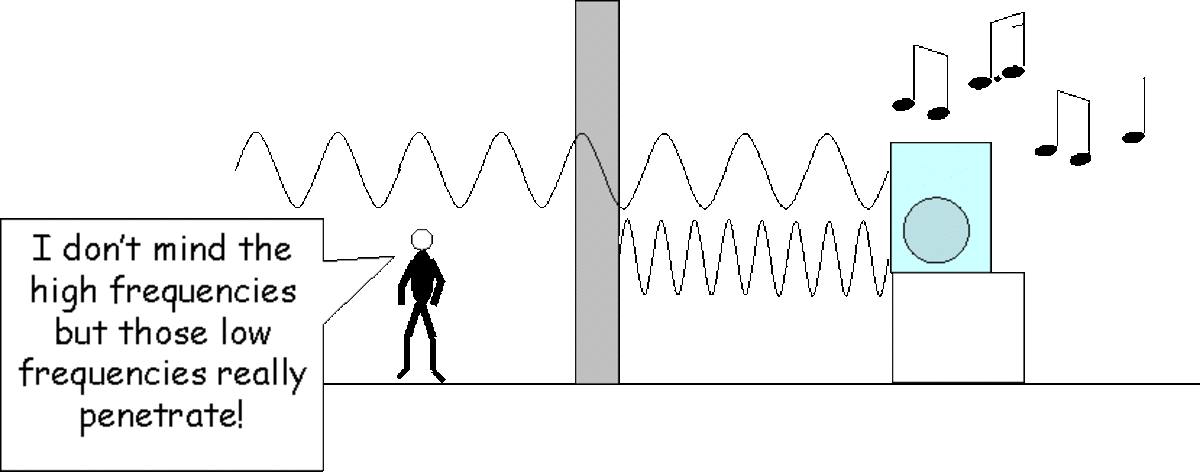Sound waves can be absorbed by objects. Higher frequencies (lower wavelengths) are absorbed more than lower frequencies (higher wavelengths).

We should say something about the ear. The human ear can detect frequencies from about 20 to 20000Hz. Anything lower than 20Hz will not be registered as a frequency and will sound more like a variation in the loudness of the sound. Anything higher than 20000Hz will be impossible for the ear to interpret. Moreover it is an interesting fact that the structure of the ear drum means that although it oscillates at the same frequency as the sound wave hitting it, it will be slightly modified. You may therefore be surprised that what you think you are hearing is not exactly the sound that is impinging on your ear.

## Pure Tones and Harmonics

When a sound is made by a musical instrument there exists a characteristic frequency known as the fundamental or first harmonic. This frequency is used to characterise which note of the scale it belongs to. The fundamental will oscillate in a sinusoidal fashion. This means that the instrument (and the air particles which are caused to vibrate in turn by the instrument) will vibrate in a sinusoidal way. For example if we were to look at an air particles displacement as a function of time it would like a sine wave. A sine wave will cause a sound known as a pure tone.

To explain a sinusoid imagine a wheel turning. Now imagine that someone has put a small mark on the outside edge of the wheel. If you now look at the wheel spinning from the end then the vertical position of the mark plotted against time will give a sine wave.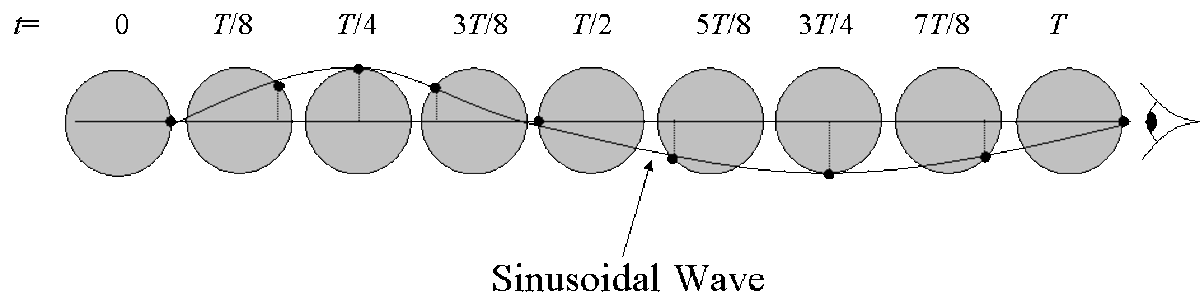A sinusoidal wave is produced by the vertical position of a mark on a spinning wheel.

There is probably no single instrument that gives out a pure tone i.e. one fundamental frequency. The instrument will produce other frequencies too. These emitted frequencies will depend on the structure of the instrument but many of them belong to a harmonic series. What is a harmonic series? If we take the fundamental frequency and double it we produce the second harmonic. If we now triple it we produce the third harmonic etc. Musical instruments will produce not only the fundamental but many harmonics too. All of these harmonics are also sinusoids. However, since there are many harmonics added together this changes the characteristics of the wave produced by the instrument. The result of adding all the harmonics up will not be a sine wave. It is interesting to note that one object that does produce a sine wave with only the first harmonic, i.e. a pure tone, is a tuning fork.A schematic showing a wave with a harmonic series going up to the fourth harmonic. Each harmonic will have integer multilples of the frequency of the fundamental or first harmonic.

## Music - What is it?

Most people know that music is not just sound. So exactly what is it that distinguishes music from mere noise?

Firstly we should discuss the frequencies involved in music. The human ear can detect frequencies between 20 and 20000Hz. Music, however, only spans the range of frequencies between 20 and 4000Hz. The piano keyboard covers pretty much all of this range; the human voice can cover 60 to 1000Hz whilst the violin can cover 200Hz to 1500Hz.

Another reason why music is different to just any other sound is due to the frequencies it uses within the range. Music chooses discrete frequencies. Let us take that example of a piano. When you press a key you cause a string to vibrate at a characteristic frequency. Move to a neighbouring key and you can sound another frequency. Without having access to the strings you are stuck with these frequencies; you cannot sound a frequency inbetween the two you have chosen. The situation is similar with a tuned guitar. If you press a string behind a fret you will cause the string to vibrate at a particular frequency. Move to the next fret and it will vibrate at another frequency. You cannot choose a frequency between the two unless you bend the string or retune the guitar. With some other instruments you can choose a continuous range of frequencies. Examples include the violin, cello and of course the human voice. Even on the guitar many players will bend strings to obtain other frequencies which usually correspond to notes on the scale but could be inbetween. However this is not an argument against the choosing of particular frequencies. A violinist must know how to choose the correct position along the finger board, however, for it to sound like music. A guitarist must also know is instrument well to be able to bend strings to obtain other notes. In other words music consists of defined discrete frequencies.

## Musical Frequencies - The Musical Ladder

So we now know that music consists of discrete frequencies. How does one choose the frequencies? Is there a fundamental natural law that governs the relationships between each frequency? For hundreds of years this debate involved the greatest minds from Pythagoras to Descartes.

The problem can be described as follows. Choose a frequency. Now to choose the next frequency one can double the frequency. If we now play both of these notes together they will go together very nicely. This is thought to be due to the fact that both notes share harmonics. For example if the first frequency has higher harmonics these will consist of twice the frequency (the second harmonic), three times the frequency (the third harmonic) and so on. The second harmonic of the first frequency matches the fundamental of the second frequency. The difference between the two frequencies is known as an octave. Now how do we choose another frequency? The next best frequency is three halves the frequency of the first one. If we play the first chosen note with this third chosen note they also sound nice. In the same way as the octave interval due to the simple ratio between both notes they will share harmonics. The interval is known as the perfect fifth. These two intervals i.e. the octave and the perfect fifth seem to produce the nicest sounds. At first glance we have started off our musical scale quite nicely but it only has three notes or frequencies.

We are still a while from forming a musical scale. One way of choosing the rest of the notes to go inbetween the octave (i.e. between the 1st and 2nd notes) is by basing the scale on perfect fifths. This is called the pythagorean method. We now choose the next note by taking three halves the frequency of the third note. Since the frequency is more than the octave (i.e. more than twice the frequency of the first note) we halve it so it is between the first and second note. We do this because we know that dividing or multiplying by two a frequency creates a nice musical interval. The next note is done the same way we take three halves the frequency of the fourth note. This time we do not halve it since it is between the first and second note. We now keep repeating this keeping the frequencies between that of the first and second notes. After doing this operation twelve times we get to a frequency slightly above two (2.027). This is actually the closest we can get to two by performing this operation. Now we have problems. The two best intervals i.e. having a ratio of one to two between frequencies and having a ratio of three to two between them are actually incompatible with each other. This method of forming a scale of twelve notes is known as just temperament. There are other methods of forming just tempered scales. The basic idea is to have a scale which can be described by simple fractions. The argument here being that notes which go well with each other will have simple relationships with each other since they will share harmonics. Whichever way you choose to form a scale based on just temperament you will always end up with a similar problem as with the pythagorean method. The simplicity of the octave and the perfect fifth fight against each other.

Many philosophers and scientists debated about this problem for hundreds of years. Galileo Galilei was one of the first of these to advocate a new form of tuning known as equal temperament to avoid the problems with just temperament. In equal temperament the octave is divided into twelve geometrically equal ratios. If this sounds strange it means that you can multiply the first note by a number to get the second and then multilply the result by the same number to get the third and so on up until the twelfth note you will get a frequency that is exactly twice the first note. The magic number is 1.0594630944. You can test this yourself. Multiply this number by itself twelve times and you will get 2 or, for the mathematically minded, calculate 1.0594630944 to the power of 12. This method of dividing the scale caused major controversy and yet it is what most of today's modern music is based on.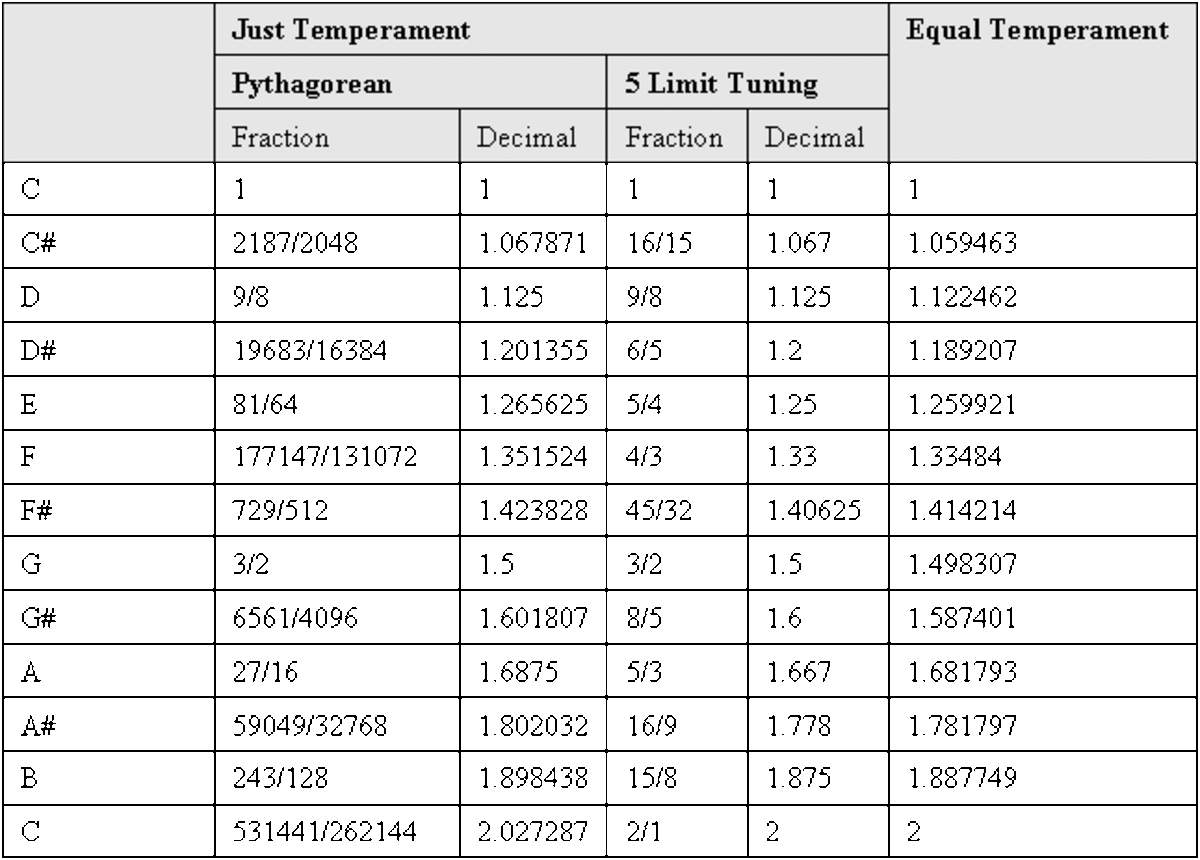Table showing how scales based on just temperament compare with those of equal temperament (for scales of 12 notes). The note is shown in the left hand column. Two different ways of producing just temperament scales are shown.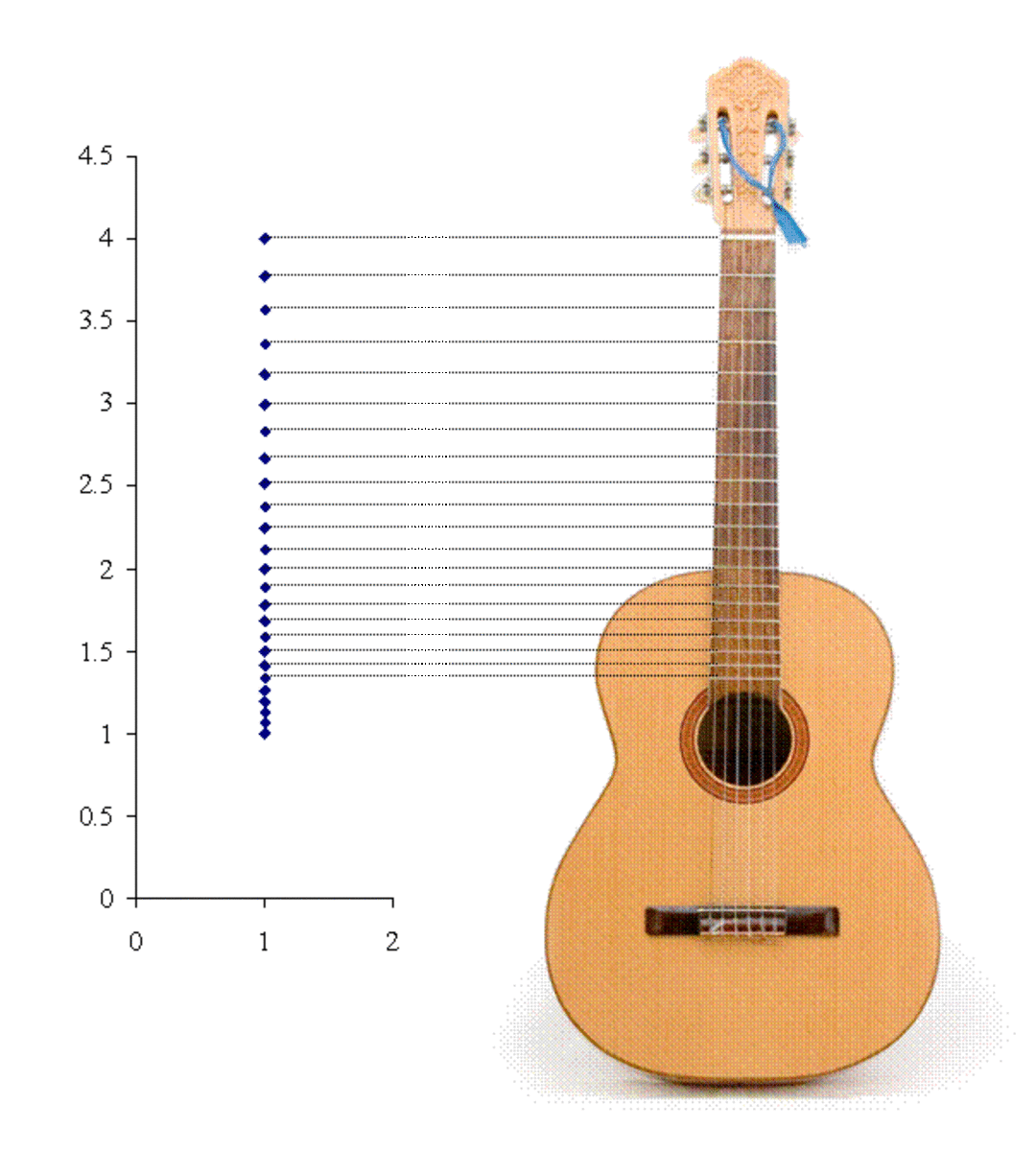In this image a guitar is shown next to a series of horizontal lines. Their vertical positions correspond to those of equal temperament.

It seems today that the equal tempered scale must have solved the problems associated with the just tempered scale. However, in equal temperament, the only interval with a simple relationship is the octave i.e. twice the frequency. There is no perfect fifth. Nevertheless the ear seems to cope with this problem. If we look at the frequency ratios in the table above we notice that the differences between the value of just and equal temperament are quite small. For example the perfect fifth which has an interval of 1.5 in just temperament has a value of 1.498307 in equal temperament.

The above details how to produce a scale with 12 notes. There are other types of scales which divide the octave into other numbers of notes. However, having 12 notes in the octave is pretty much the staple of most western music. One possibility for this may be that although the perfect fifth interval of 1.5 does not exist in equal temperament the equivalent interval is very close when you geometrically divide the scale (into twelve equal parts). It is sometimes hard to believe that nearly all of the music we hear is based on just these 12 notes. It must be noted that the type of music we hear is heavily dependent on which of these notes are actually used. This is dictated by the key that the music is played in.

## Scales Based on the 12 Note Chromatic Scale

The twelve notes of the chromatic scale are rarely all used in one piece of music. The key of the music is chosen such that only a few of the notes are used such that intervals in the scale are not just semitones (the difference between neighbouring notes in the chromatic scale) but also tones (double a semitone) and sometimes more. This turns out to be very important to the feel of the music. The major scale, for example, has seven different notes based on successive intervals of tone, tone, semitone, tone, tone, tone and semitone. There are however many other scales. Minor scales, based on different intervals tend to sound more sombre. or sometimes reminiscent of music from the East. Much popular music is based on blues pentatonic scales. As the name suggests there are five notes in this scale.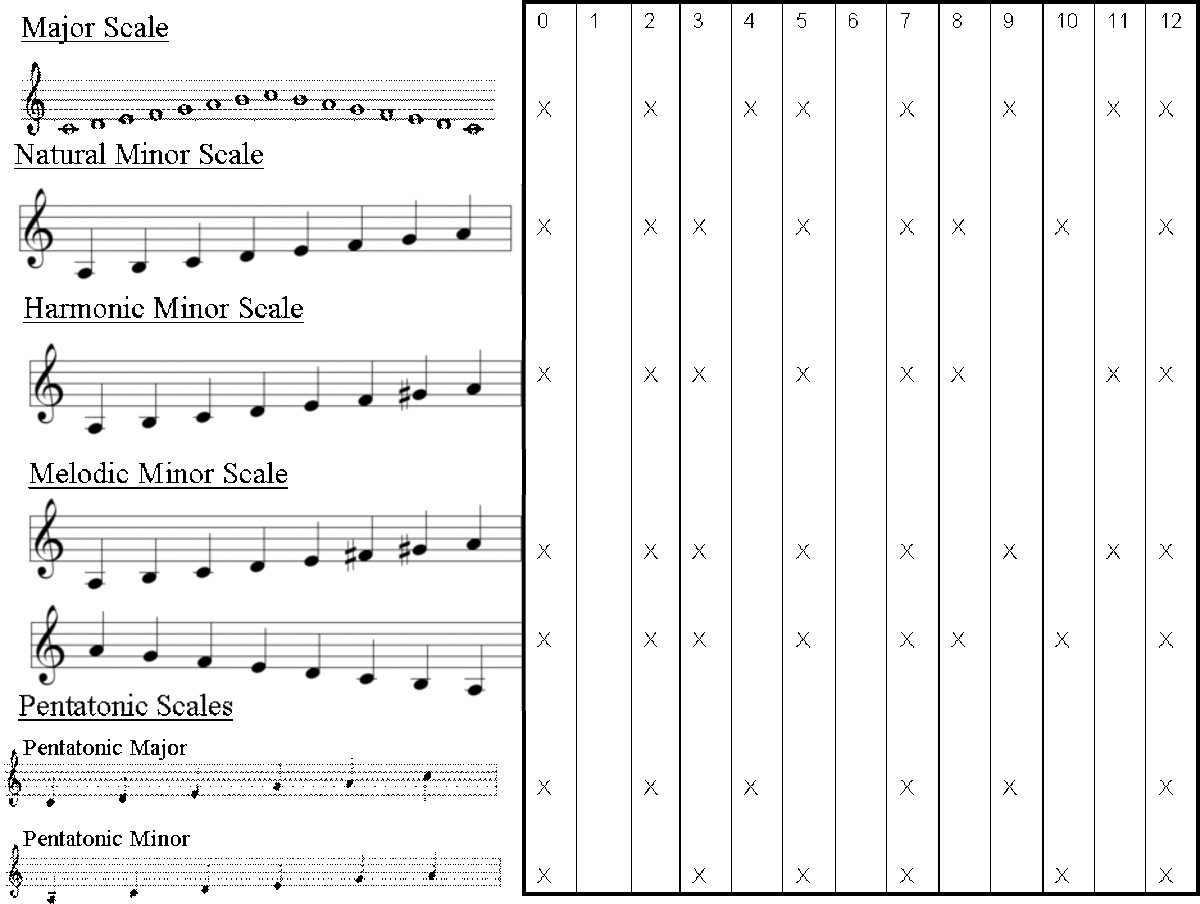Schematic showing the notes chosen by different keys. The columns represent each of the 12 notes of the chromatic scale. The difference between neighbouring notes in the chromatic scale is known as a semitone.

## Musical Instruments - Strings and Pipes

Most musical instruments consist of strings and pipes. When a string is plucked, or a pipe is blown into, a travelling wave goes to the end of the string or pipe. The wave is reflected at the end such that there are now two waves: There is still a component of the wave travelling oposite to the reflected wave. Thus we have two waves, exactly the same, but travelling in opposite directions. These two waves add together to form a standing wave. This wave no longer travels down the pipe or along the string but instead just changes its amplitude.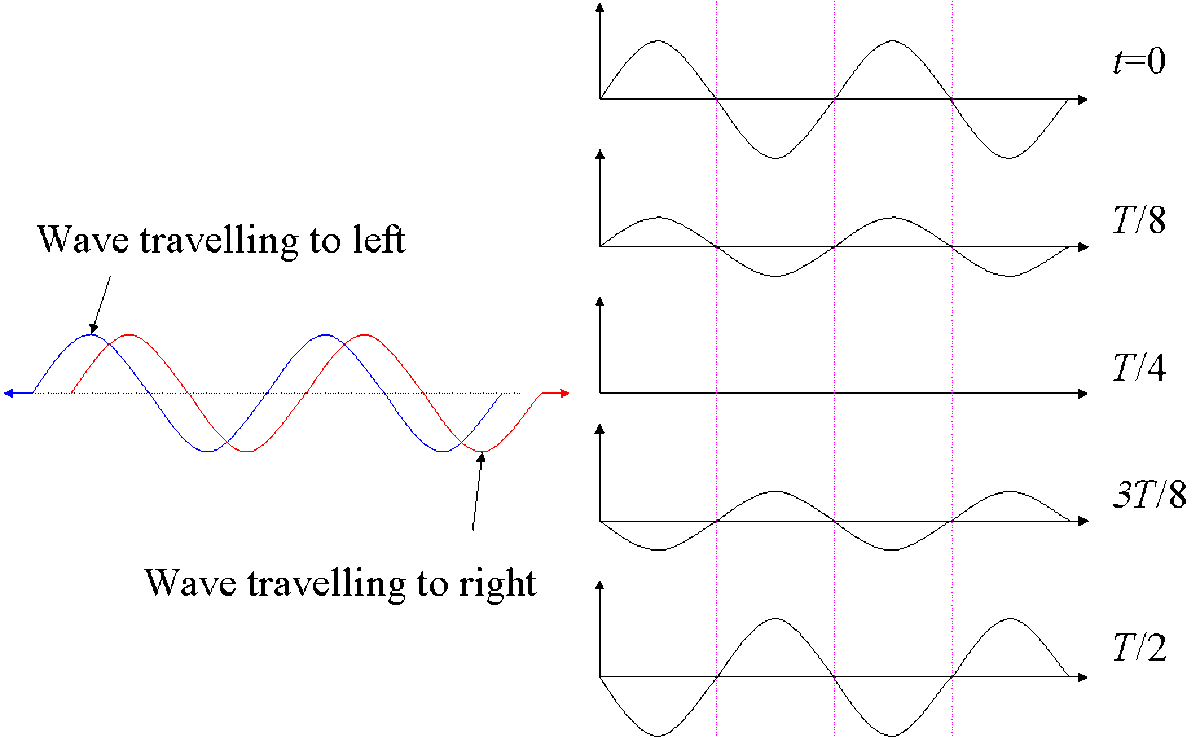This schematic demonstrates how a standing wave is formed by two waves which have exactly equal properties except that they are travelling in opposite directions. Four snapshots of the standing wave are shown (T represents the period).

Shortly after a string is plucked or air is blown down a pipe the travelling waves travel up and down forming the standing wave. Many waves with different frequencies are initially formed but many, that do not have a harmonic relationship with the fundamental frequency, will quickly decay in amplitude. The string or pipe will quickly settle to vibrating at its fundamental frequency with its own harmonic series.

Standing waves on strings must not move at the ends since these are fixed. This is a very important statement since the length of the string will determine the wavelength of the sound produced. In the figure is shown some of the standing waves that are allowed to form on a stretched string.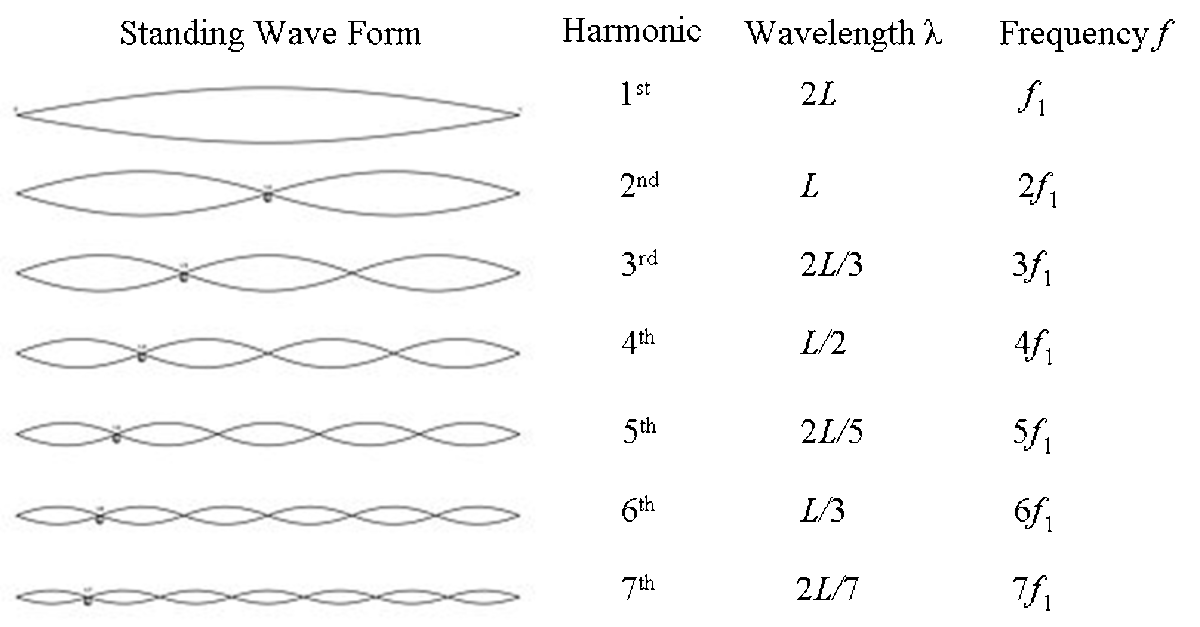Schematic showing the harmonics series that allowed on a stretched string. All harmonics are allowed.

In addition to the allowed harmonics two other properties are needed to describe the frequency of the vibrating string: the tension in the string and the mass of the string. If we pull a string tighter the stored energy in the string increases. Thus when we pluck it it will vibrate faster and emit a higher frequency sound wave. Similarly if we release tension the frequency of the vibration decreases. If the string is heavy it will also vibrate at a lower frequency than if the string is light. This is due to inertia. Heavier objects will move slower than lighter objects when the same force is applied. The force here is the tension in the string. So, in summary, if we know the tension in the string, the mass of the string and the length of the string we can calculate the frequency (and wavelength, period etc) of the first harmonic or fundamental.

Standing waves that are formed in pipes are more complicated to describe than those on strings. The principle difference, however, is that pipes can be open at both ends or open at one end and closed at the other. Since the air in a pipe moves parallel to the pipe structure the air cannot vibrate at a closed end. At an open end the particles vibrate with maximum amplitude. The figure below shows that if the pipe is open at both ends all the harmonics are allowed. If the pipe is open only at one end then only the odd harmonics, i.e. multiples or the fundamental frequency which are odd numbers, are allowed.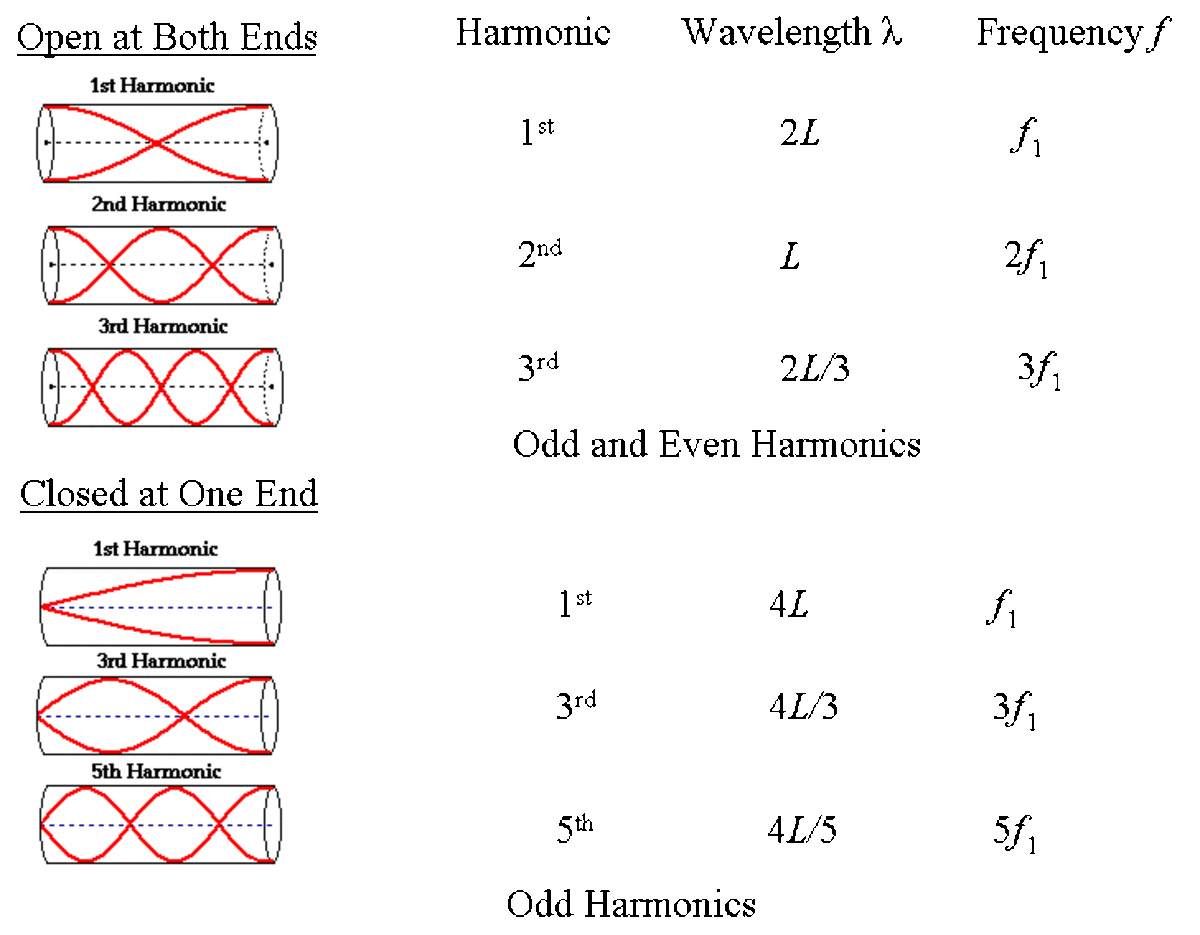The diagram demonstrates how standing wave harmonics are formed in pipes. If the pipe is open at both ends all harmonics are allowed (1st, 2nd, 3rd etc.). If the pipe is closed at one end then only odd harmonics are allowed (1st, 3rd, 5th etc.).

In addition to knowing the length of the pipe and knowing whether it is open at both ends or closed at one end more is needed to calculate the frequency of the first harmonic. The speed of sound in air is needed. This can be calculated if one knows the air pressure and the mass or air molecules. This can be compared to the properties of tension and mass in a vibrating string. If the air pressure is increased there is more stored energy so the sound wave velocity (and frequency) will increase. The larger the mass of the molecules the lower the velocity (and frequency) due to inertia.

## Musical Instruments and Timbre

There are many different types of musical instruments but why. There are some obvious advantages to being able to have access to several notes at the same time such as a piano or guitar. Then again to get a continuous note such as on a woodwind instrument or violin is also an advantage. Yes this is important. However, if you play middle C on a piano and middle C on a violin, although you are playing the same note, they somehow sound different. This is all down to a property known as timbre. In short the timbre is the resultant sound from all the harmonics of the particular sound.

When a string or pipe starts producing the harmonics, the harmonics will, in turn, transmit the vibrations (the allowed harmonics) to the rest of the instrument. The rest of the instrument will start to resonate at some of these frequencies. The instruments structure then chooses which harmonics it prefers i.e. which will be strong, which will be weak etc. The shape of the instrument is very important  It is the collection of resultant harmonics that gives each instrument its characteristic sound and timbre.

To demonstrate this below is shown the first and second harmonics on the left. On the right is the result of adding them together. The vertical line is the period of the first harmonic. The resultant wave still has the same frequency as the 1st harmonic but the waveform is different. The note of the resultant will have the same pitch or frequency as the first harmonic (a pure harmonic sound can be produced from a tuning fork) but the form is different. The first harmonic and resultant therefore sound different.

The figure below shows the result of adding together all the odd harmonics. This could be the simplified case of a pipe which is closed at one end (see above - a pipe that is closed at one end is only allowed to have odd harmonics). Once again the resultant is the same period as the first harmonic. The forms of the resultant and the first harmonic are very different; they will thus sound very different but will still have the same frequency.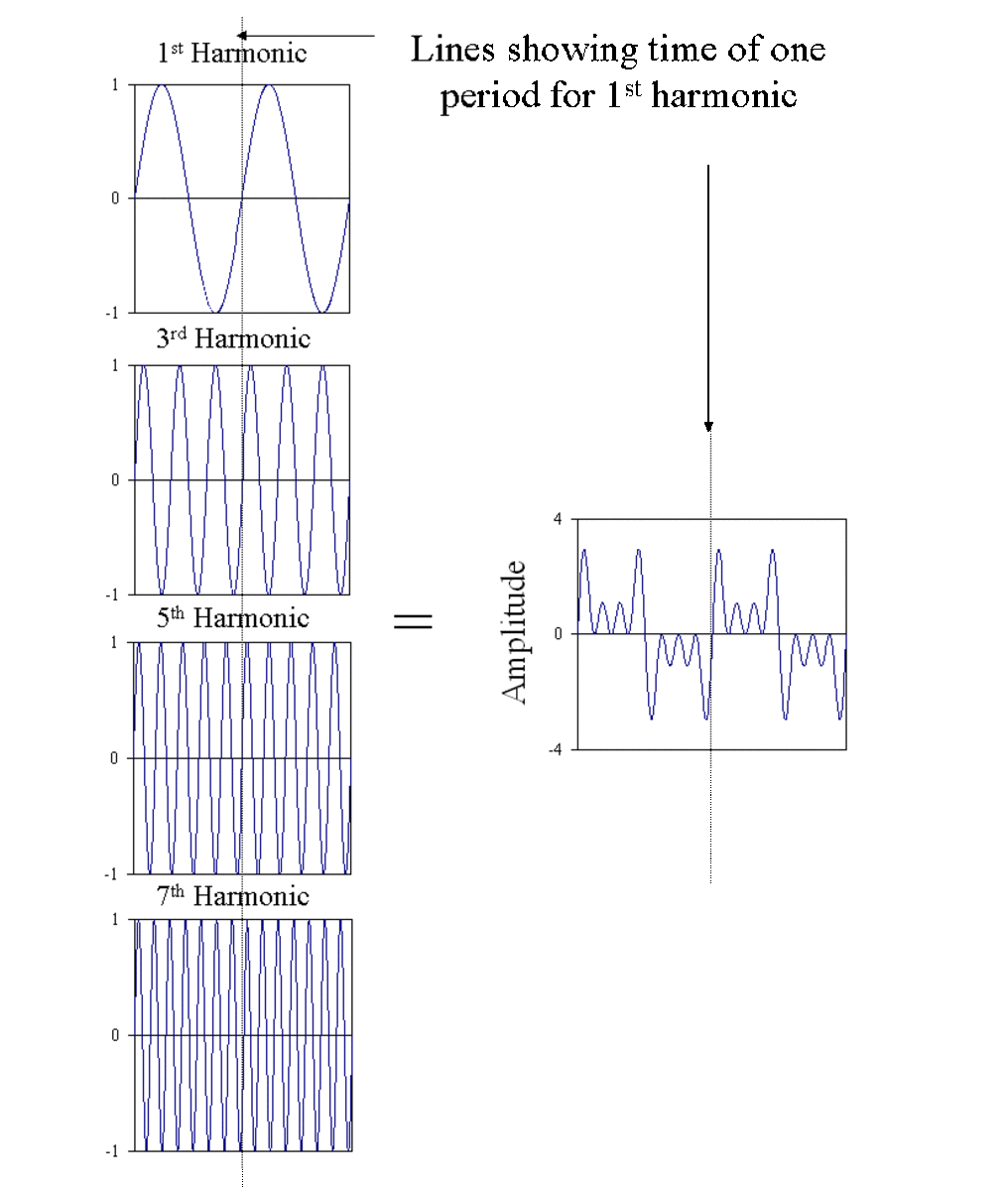These graphs show the odd harmonics up to the seventh and the result of adding them together.

For completeness the diagram below shows the result of adding all the even harmonics together.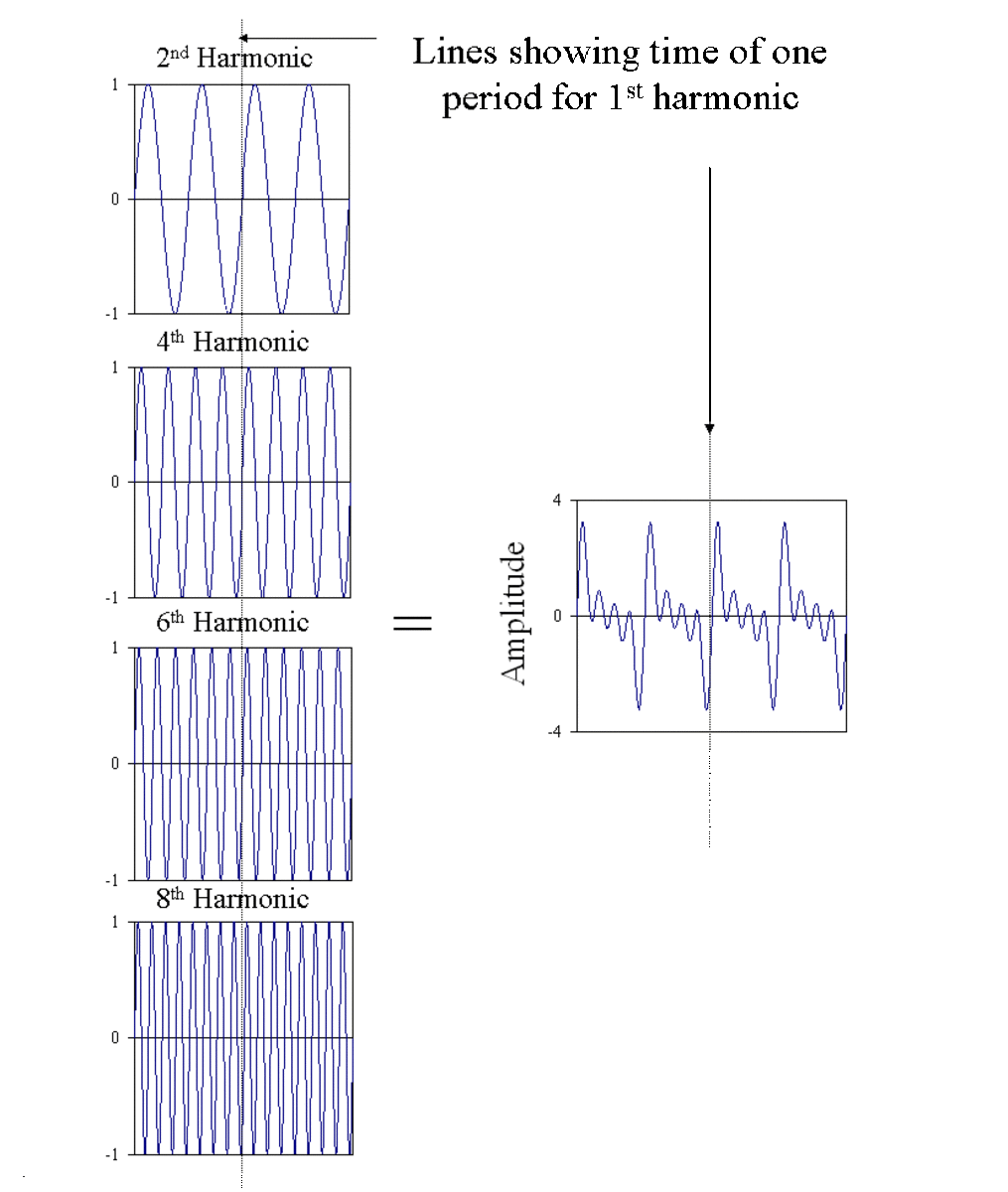These graphs show the even harmonics up to the eighth and the result of adding them together.

The above diagrams showing the result of adding together harmonics are very simplified. The waveforms from real instruments are more complicated. Below are shown examples from the flute, clarinet, oboe and saxophone. Although all four waveforms look very different they all repeat i.e. they are periodic. The period will be the same as the fundamental or first harmonic.